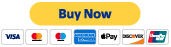top of page

## Price Calculator

The pricing below is for illustration purposes only.  Exact quilt measurments will need to be provided.  To calculate square inches:

Multiply length × width. Simply multiply your measurements for length and width to determine the area of your square or rectangular area in square inches. For example, let's say that your quilt is 65" wide x 88" long,  In this case, your quilt is 5,720 square inches.bottom of page